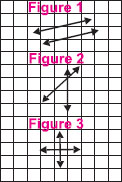# User ForumSubject :IMO    Class : Class 4

Study the given graph.
Which of the given figures show pair of intersecting lines?A Figure 1 and 2
B Figure 2 and 3
C Figure 1 and 3
D None of these

figure 3 is a perpendicular line so the answer is d

Class : Class 4
B

Class : Class 5